# Non-Verbal Reasoning (Analogy)

Analogy means relationship.  Let us have a look at an example:
Teacher : Pen  : : Soldier : _________
What should come in the blank? If teacher's main tool is pen, Soldier's main tool is a gun.
Similarly, we have to identify the relationship between in figures A and B so that to identify the option which got relationship with figure C.
Just look at few examples and you can easily understand these problems:

1.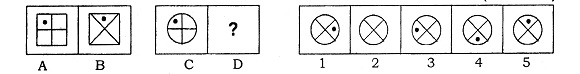The square in PF(A) rotated 90$^0$ clockwise along with dot.  So option 5 is correct.

2.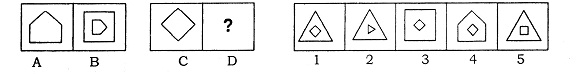Pentagon in PF(A) became small and circumscribed with Square in PF(B).  So If a square has to become small and to be circumscribed with triangle.  So option 1 is correct.  option 5 is rules out as the square rotated 45$^0$ instead of 90$^0$.

3.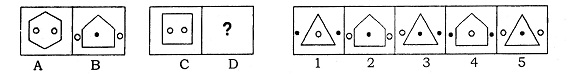Here the hexagon becae pentagon and the dots came out of the diagram, and a new darkened dot appeared in the middle.  So PF(C) should become triangle and two dots must come out and a darkened dot must appear in the iddle.  So correct Option 5

4.Here Bottom square became big, and the figure above it, came into it and pentagon appeared in the triangle.  So in PF(C) pentagon must become big, and square must be inside it and a hexagon should appear in it.  so correct option 2

5.Simple one.  Two circles became a single square, and the square became two squares. So two triangles must become single triangle and circle must become two circles. So answer option 4.

6.Another simple one.  the directions of the arrows changed their positions.  So answer option 4.

7.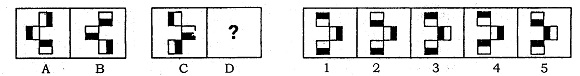In PF(1), top half darkened rectangle turned 90$^0$ clockwise, middle half darkened rectangle turned anti-clockwise 90$^0$ and bottom half darkened rectangle turned clockwise by 90$^0$.  So turn the rectangles in PF(C), clockwise, anti-clockwise, and clockwise. So correct Option 1

8.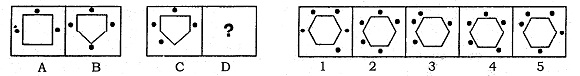In PF(A), the square has three dots each at the middle of its sides.  In PF(B), square became pentagon, and number of dots got increased by one and one dot occupied the vertex.  So PF(C) must become hexagon and there must be 5 dots and one dot should occupy the vertex. Correct option 4

9.The entire diagnol rotated by about 180$^0$ clock wise and the open circle became darkened.  So the square must be darkened and the entire diagnol should rotate by 180$^0$.  So correct option 3

10.Simple observation.  Pentagon at the top became bigger and square came inside of it.  So hexagon in PF(C) should become big and circle should enter into it.  So correct option 3

Questions for Practice
11.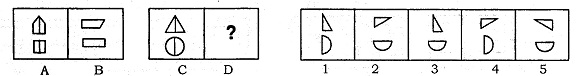12.13.14.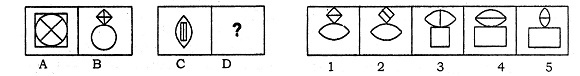15.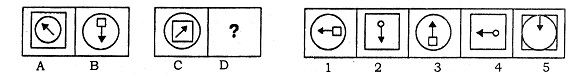16.17.18.19.20.21.